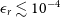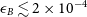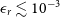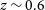Hostname: page-component-cd4964975-g4d8c Total loading time: 0 Render date: 2023-04-02T10:37:01.696Z Has data issue: true Feature Flags: { "useRatesEcommerce": false } hasContentIssue true

# Early-time searches for coherent radio emission from short GRBs with the Murchison Widefield Array

Published online by Cambridge University Press:  03 February 2022

*
Corresponding author: J. Tian, email: jun.tian@postgrad.curtin.edu.au

## Abstract

Many short gamma-ray bursts (GRBs) originate from binary neutron star mergers, and there are several theories that predict the production of coherent, prompt radio signals either prior, during, or shortly following the merger, as well as persistent pulsar-like emission from the spin-down of a magnetar remnant. Here we present a low frequency (170–200 MHz) search for coherent radio emission associated with nine short GRBs detected by the Swift and/or Fermi satellites using the Murchison Widefield Array (MWA) rapid-response observing mode. The MWA began observing these events within 30–60 s of their high-energy detection, enabling us to capture any dispersion delayed signals emitted by short GRBs for a typical range of redshifts. We conducted transient searches at the GRB positions on timescales of 5 s, 30 s, and 2 min, resulting in the most constraining flux density limits on any associated transient of 0.42, 0.29, and 0.084 Jy, respectively. We also searched for dispersed signals at a temporal and spectral resolution of 0.5 s and 1.28 MHz, but none were detected. However, the fluence limit of 80–100 Jy ms derived for GRB 190627A is the most stringent to date for a short GRB. Assuming the formation of a stable magnetar for this GRB, we compared the fluence and persistent emission limits to short GRB coherent emission models, placing constraints on key parameters including the radio emission efficiency of the nearly merged neutron stars ($\epsilon_r\lesssim10^{-4}$ ), the fraction of magnetic energy in the GRB jet ($\epsilon_B\lesssim2\times10^{-4}$ ), and the radio emission efficiency of the magnetar remnant ($\epsilon_r\lesssim10^{-3}$ ). Comparing the limits derived for our full GRB sample (along with those in the literature) to the same emission models, we demonstrate that our fluence limits only place weak constraints on the prompt emission predicted from the interaction between the relativistic GRB jet and the interstellar medium for a subset of magnetar parameters. However, the 30-min flux density limits were sensitive enough to theoretically detect the persistent radio emission from magnetar remnants up to a redshift of$z\sim0.6$ . Our non-detection of this emission could imply that some GRBs in the sample were not genuinely short or did not result from a binary neutron star merger, the GRBs were at high redshifts, these mergers formed atypical magnetars, the radiation beams of the magnetar remnants were pointing away from Earth, or the majority did not form magnetars but rather collapse directly into black holes.

## Keywords

Type
Research Article
© The Author(s), 2022. Published by Cambridge University Press on behalf of the Astronomical Society of Australia

## Access options

Get access to the full version of this content by using one of the access options below. (Log in options will check for institutional or personal access. Content may require purchase if you do not have access.)

## References

Abbott, B. P., et al. 2017, PhRvL, 119, 161101Google Scholar
Abbott, B.P. et al. 2020, LRR, 23, 3Google Scholar
Ackermann, M., et al. 2010, ApJ, 716, 1178Google Scholar
Anderson, G. E., et al. 2018a, MNRAS, 473, 1512Google Scholar
Anderson, G. E., et al. 2021a, PASA, 38, e026Google Scholar
Anderson, G. E., et al. 2021b, MNRAS, 503, 4372Google Scholar
Anderson, M. M., et al. 2018b, ApJ, 864, 22Google Scholar
Collaboration, Astropy, et al. 2018, AJ, 156, 123 Google Scholar
Baird, G. A., et al. 1975, ApJ, 196, L11CrossRefGoogle Scholar
Bannister, K. W., Murphy, T., Gaensler, B. M., & Reynolds, J. E. 2012, ApJ, 757, 38CrossRefGoogle Scholar
Barthelmy, S. D., et al. 2005, SSR, 120, 143CrossRefGoogle Scholar
Barthelmy, S. D., et al. 2019, GRB Coordinates Network, 24899, 1Google Scholar
BÉguÉ, D., Burgess, J. M., & Greiner, J. 2017, ApJ, 851, L19CrossRefGoogle Scholar
Belczynski, K., Holz, D. E., Fryer, C. L., Berger, E., Hartmann, D. H., & O’Shea, B. 2010, ApJ, 708, 117CrossRefGoogle Scholar
Bell, M. E., et al. 2019, MNRAS, 482, 2484Google Scholar
Berger, E. 2014, ARA&A, 52, 43CrossRefGoogle Scholar
Bloom, J. S., Frail, D. A., & Sari, R. 2001, AJ, 121, 2879CrossRefGoogle Scholar
Burrows, D. N., et al. 2005, SSR, 120, 165Google Scholar
Burrows, D. N., et al. 2006, ApJ, 653, 468CrossRefGoogle Scholar
Cavallo, G., & Rees, M. J. 1978, MNRAS, 183, 359CrossRefGoogle Scholar
Cenko, S. B., et al. 2019a, GRB Coordinates Network, 25945, 1Google Scholar
Chu, Q., Howell, E. J., Rowlinson, A., Gao, H., Zhang, B., Tingay, S. J., BoËr, M., & Wen, L. 2016, MNRAS, 459, 121CrossRefGoogle Scholar
Cordes, J. M., & Lazio, T. J. W. 2002, arXiv e-prints, pp astro–ph/0207156Google Scholar
DeLaunay, J., Tohuvavohu, A., & Kennea, J. 2020a, GRB Coordinates Network, 27444, 1Google Scholar
Dessenne, C. A. C., et al. 1996, MNRAS, 281, 977CrossRefGoogle Scholar
Dewdney, P. E., Hall, P. J., Schilizzi, R. T., & Lazio, T. J. L. W. 2009, IEEE Proc., 97, 1482CrossRefGoogle Scholar
Duchesne, S. W., Johnston-Hollitt, M., Zhu, Z., Wayth, R. B., & Line, J. L. B. 2020, PASA, 37, e037CrossRefGoogle Scholar
Duncan, R. C., & Thompson, C. 1992, ApJ, 392, L9CrossRefGoogle Scholar
Evans, P. A., et al. 2009, MNRAS, 397, 1177CrossRefGoogle Scholar
Evans, P. A., et al. 2010, A&A, 519, A102Google Scholar
Farah, W., et al. 2019, MNRAS, 488, 2989Google Scholar
Fong, W., & Berger, E. 2013, ApJ, 776, 18CrossRefGoogle Scholar
Fong, W., Berger, E., Margutti, R., & Zauderer, B. A. 2015, ApJ, 815, 102CrossRefGoogle Scholar
Fong, W., et al. 2021, ApJ, 906, 127Google Scholar
Gehrels, N., et al. 2004, ApJ, 611, 1005Google Scholar
Ghumatkar, P., Sharma, V., Bhattacharya, D., Khanam, T., Vibhute, A., Vadawale, S., & AstroSat CZTI Collaboration 2019, GRB Coordinates Network, 25289, 1Google Scholar
Goldstein, A., et al. 2017, ApJ, 848, L14Google Scholar
Gompertz, B. P., Levan, A. J., & Tanvir, N. R. 2020, ApJ, 895, 58CrossRefGoogle Scholar
Gourdji, K., Rowlinson, A., Wijers, R. A. M. J., & Goldstein, A. 2020, MNRAS, 497, 3131CrossRefGoogle Scholar
Gupta, V., et al. 2021, MNRAS, 501, 2316Google Scholar
Hancock, P. J., Hurley-Walker, N., & White, T. E. 2019a, A&C, 27, 23CrossRefGoogle Scholar
Hancock, P. J., Trott, C. M., & Hurley-Walker, N. 2018, PASA, 35, e011CrossRefGoogle Scholar
Hancock, P. J., et al. 2019b, PASA, 36, e046Google Scholar
Hunter, J. D. 2007, CSE, 9, 90 CrossRefGoogle Scholar
Hurley-Walker, N., & Hancock, P. J. 2018, A&C, 25, 94CrossRefGoogle Scholar
Hurley-Walker, N., et al. 2017, MNRAS, 464, 1146Google Scholar
Hurley, K., et al. 2013, ApJS, 207, 39CrossRefGoogle Scholar
Inoue, T., Asano, K., & Ioka, K. 2011, ApJ, 734, 77CrossRefGoogle Scholar
James, C. W., Anderson, G. E., Wen, L., Bosveld, J., Chu, Q., Kovalam, M., Slaven-Blair, T. J., & Williams, A. 2019, MNRAS, 489, L75CrossRefGoogle Scholar
Japelj, J., et al. 2019, GRB Coordinates Network, 24916, 1Google Scholar
Jones, E., et al. 2001, SciPy: Open Source Scientific Tools for Python, ://www.scipy.org/Google Scholar
Kann, D. A. 2013, in EAS Publications Series, Vol. 61, EAS Publications Series, ed. A. J. Castro-Tirado, J. Gorosabel, & I. H. Park, 309 (arXiv:1212.0040), 10.1051/eas/1361049CrossRefGoogle Scholar
Kaplan, D. L., et al. 2015, ApJ, 814, L25CrossRefGoogle Scholar
Kaplan, D. L., Murphy, T., Rowlinson, A., Croft, S. D., Wayth, R. B., & Trott, C. M. 2016, PASA, 33, e050CrossRefGoogle Scholar
Katz, J. I. 1997, ApJ, 490, 633CrossRefGoogle Scholar
Kouveliotou, C., Meegan, C. A., Fishman, G. J., Bhat, N. P., Briggs, M. S., Koshut, T. M., Paciesas, W. S., & Pendleton, G. N. 1993, ApJ, 413, L101CrossRefGoogle Scholar
Kurtzer, G. M., Sochat, V., & Bauer, M. W. 2017, PLOS ONE, 12, e0177459 CrossRefGoogle Scholar
Lasky, P. D., Haskell, B., Ravi, V., Howell, E. J., & Coward, D. M. 2014, PhRvD, 89, 047302CrossRefGoogle Scholar
Lattimer, J. M. 2012, ARNPS, 62, 485CrossRefGoogle Scholar
Lien, A., et al. 2016, ApJ, 829, 7Google Scholar
Lipunov, V. M., & Panchenko, I. E. 1996, A&A, 312, 937 Google Scholar
, H.-J., Liang, E.-W., Zhang, B.-B., & Zhang, B. 2010, ApJ, 725, 1965CrossRefGoogle Scholar
Lucek, S. G., & Bell, A. R. 2000, MNRAS, 314, 65CrossRefGoogle Scholar
Lyutikov, M. 2013, ApJ, 768, 63CrossRefGoogle Scholar
Macquart, J. P., et al. 2020, Nat, 581, 391CrossRefGoogle Scholar
Mailyan, B., & Meegan, C. 2019, GRB Coordinates Network, 25636, 1Google Scholar
Medvedev, M. V., Fiore, M., Fonseca, R. A., Silva, L. O., & Mori, W. B. 2005, ApJ, 618, L75CrossRefGoogle Scholar
Meegan, C., et al. 2009, ApJ, 702, 791CrossRefGoogle Scholar
Metzger, B. D., Berger, E., & Margalit, B. 2017, ApJ, 841, 14CrossRefGoogle Scholar
Metzger, B. D., & Zivancev, C. 2016, MNRAS, 461, 4435 CrossRefGoogle Scholar
MilosavljeviĆ, M., & Nakar, E. 2006, ApJ, 651, 979CrossRefGoogle Scholar
Mizuno, Y., Pohl, M., Niemiec, J., Zhang, B., Nishikawa, K.-I., & Hardee, P. E. 2011, ApJ, 726, 62CrossRefGoogle Scholar
Narayan, R., Paczynski, B., & Piran, T. 1992, ApJ, 395, L83CrossRefGoogle Scholar
Narayana Bhat, P., et al. 2016, ApJS, 223, 28Google Scholar
Obenberger, K. S., et al. 2014, ApJ, 785, 27CrossRefGoogle Scholar
Ochsenbein, F., Bauer, P., & Marcout, J. 2000, A&AS, 143, 23CrossRefGoogle Scholar
Offringa, A. R., & Smirnov, O. 2017, MNRAS, 471, 301CrossRefGoogle Scholar
Offringa, A. R., et al. 2014, MNRAS, 444, 606Google Scholar
Palaniswamy, D., Wayth, R. B., Trott, C. M., McCallum, J. N., Tingay, S. J., & Reynolds, C. 2014, ApJ, 790, 63CrossRefGoogle Scholar
Panaitescu, A. 2005, MNRAS, 363, 1409CrossRefGoogle Scholar
Panaitescu, A., & Kumar, P. 2002, ApJ, 571, 779CrossRefGoogle Scholar
Rees, M. J., & Meszaros, P. 1992, MNRAS, 258, 41CrossRefGoogle Scholar
Rezzolla, L., Giacomazzo, B., Baiotti, L., Granot, J., Kouveliotou, C., & Aloy, M. A. 2011, ApJ, 732, L6CrossRefGoogle Scholar
Rowlinson, A., & Anderson, G. E. 2019, MNRAS, 489, 3316CrossRefGoogle Scholar
Rowlinson, A., et al. 2010, MNRAS, 409, 531Google Scholar
Rowlinson, A., O’Brien, P. T., Metzger, B. D., Tanvir, N. R., & Levan, A. J. 2013, MNRAS, 430, 1061CrossRefGoogle Scholar
Rowlinson, A., et al. 2019, MNRAS, 490, 3483CrossRefGoogle Scholar
Rowlinson, A., et al. 2020, arXiv e-prints, p. arXiv:2008.12657Google Scholar
Rowlinson, A., et al. 2021, MNRAS, 506, 5268CrossRefGoogle Scholar
Sakamoto, T., et al. 2019, GRB Coordinates Network, 25953, 1Google Scholar
Santana, R., Barniol Duran, R., & Kumar, P. 2014, ApJ, 785, 29CrossRefGoogle Scholar
Sarin, N., Lasky, P. D., & Ashton, G. 2020, MNRAS, 499, 5986CrossRefGoogle Scholar
Seaman, R., et al. 2011, Sky Event Reporting Metadata Version 2.0, IVOA Recommendation 11 July 2011 (arXiv:1110.0523), 10.5479/ADS/bib/2011ivoa.spec.0711SGoogle Scholar
Soderberg, A. M., et al. 2006, ApJ, 650, 261CrossRefGoogle Scholar
Sokolowski, M., et al. 2017, PASA, 34, e062Google Scholar
Sokolowski, M., et al. 2021, PASA, 38, e023Google Scholar
Sonbas, E., et al. 2019a, GRB Coordinates Network, 24888, 1Google Scholar
Spergel, D. N., et al. 2003, ApJS, 148, 175Google Scholar
Staley, T. D., & Fender, R. 2016, arXiv e-prints, p. arXiv:1606.03735Google Scholar
Svinkin, D., et al. 2017, GRB Coordinates Network, 21735, 1Google Scholar
Swinbank, J. 2014, A&C, 7, 12CrossRefGoogle Scholar
Taylor, M. B. 2005, in Astronomical Society of the Pacific Conference Series, Vol. 347, Astronomical Data Analysis Software and Systems XIV, ed. Shopbell, P., Britton, M., & Ebert, R., 29 Google Scholar
Taylor, J. H., Manchester, R. N., & Lyne, A. G. 1993, ApJS, 88, 529CrossRefGoogle Scholar
The Astropy Collaboration, et al. 2013, A&A, 558, 9 Google Scholar
Thompson, C. 1994, MNRAS, 270, 480CrossRefGoogle Scholar
Tingay, S. J., et al. 2013, PASA, 30, e007Google Scholar
Totani, T. 2013, PASJ, 65, L12CrossRefGoogle Scholar
Tremblay, S. E., et al. 2015, PASA, 32, e005Google Scholar
Usov, V. V. 1992, Nat, 357, 472CrossRefGoogle Scholar
Usov, V. V. 1994, MNRAS, 267, 1035CrossRefGoogle Scholar
Usov, V. V., & Katz, J. I. 2000, A&A, 364, 655Google Scholar
van der Horst, A. J., et al. 2008, A&A, 480, 35CrossRefGoogle Scholar
van der Walt, S., Colbert, S. C., & Varoquaux, G. 2011, CSE, 13, 22 CrossRefGoogle Scholar
van Haarlem, M. P., et al. 2013, A&A, 556, A2Google Scholar
Veres, P., Hui, C. M., Meegan, C., Wood, J., & Fermi GBM Team 2020a, GRB Coordinates Network, 27453, 1Google Scholar
Veres, P., Meegan, C., & Fermi GBM Team 2020b, GRB Coordinates Network, 27466, 1Google Scholar
Wayth, R. B., et al. 2018, PASA, 35, 33CrossRefGoogle Scholar
Wenger, M., et al. 2000, A&AS, 143, 9Google Scholar
Wright, E. L. 2006, PASP, 118, 1711CrossRefGoogle Scholar
Yao, J. M., Manchester, R. N., & Wang, N. 2017, ApJ, 835, 29CrossRefGoogle Scholar
Yost, S. A., Harrison, F. A., Sari, R., & Frail, D. A. 2003, ApJ, 597, 459CrossRefGoogle Scholar
Zhang, B. 2014, ApJ, 780, L21CrossRefGoogle Scholar
Zhang, B., & MÉszÁros, P. 2001, ApJ, 552, L35CrossRefGoogle Scholar
Zhang, B., et al. 2009, ApJ, 703, 1696CrossRefGoogle Scholar